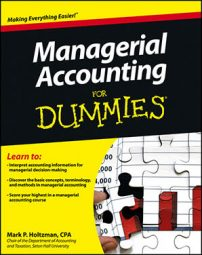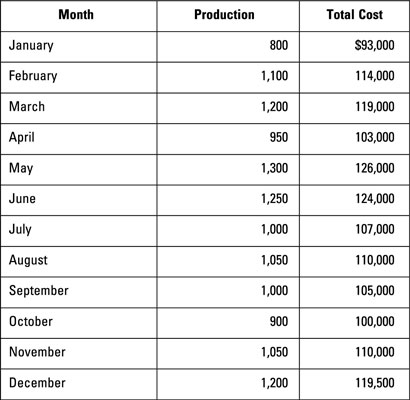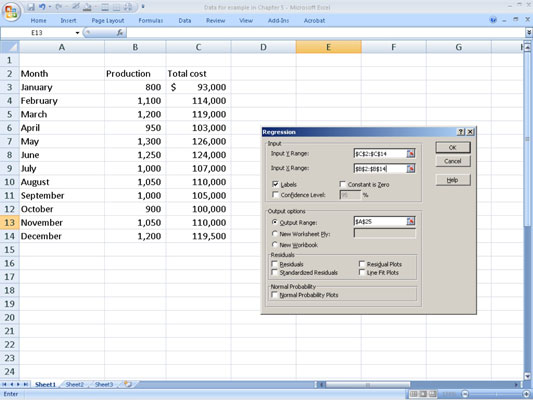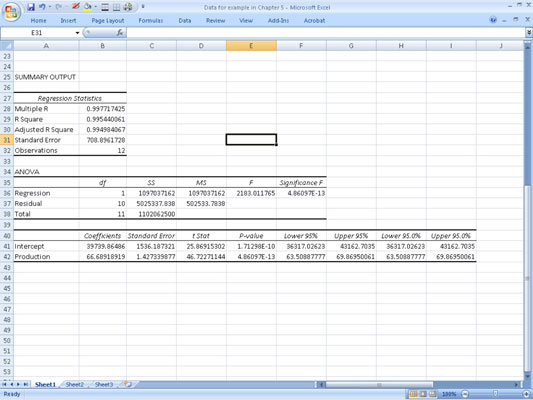##### Managerial Accounting For DummiesStatistical regression allows you to apply basic statistical techniques to estimate cost behavior. Don’t panic! Excel (or a statistical analysis package) can quickly figure this information out for you.

Before starting, make sure you’ve installed the Microsoft Office Excel Analysis ToolPak. To confirm whether you already have it, click on “Data” and look for an item in the drop-down menu that says “Data Analysis.”

If you don’t see this item, you need to install the ToolPak. Go to “Excel Options” and click on “Add-Ins.” From here, go to the “Manage” box and click on “Excel Add-ins” and then “Go.” From the “Add-Ins Available” box, click on the “Analysis ToolPak” check box and then click on “OK.”

1. Enter the data into Excel.

Create a table of data in Excel, listing each month’s production activity level and total cost.2. Run a regression analysis in Excel.

Run a regression on the data in Excel by following these steps:

1. Click on “Data.”

2. Click on “Data Analysis” and then “Regression;” then click on “OK.”

3. Under “Input Y Range,” enter the range for the total cost data, including the heading.

4. Under “Input X Range,” enter the production data, including the heading.

5. Click on the box for “Labels.”

7. Click on “OK.”When regressing total cost on a cost driver, the total cost data always goes into the “Input Y Range,” and the cost driver data always goes into the “Input X Range.”

From all the information shown in the output, you really only need two numbers. In the bottom table, look at the column marked “Coefficients.” The number labeled “Intercept” (\$39,739) is a statistical estimate of the fixed cost. The number labeled “Production” (\$66.69) gives you a statistical estimate of the variable cost per unit.Based on these regression results, you can determine that making 1,000 units would create total variable costs of \$66,690 (1,000 units x \$66.69 per unit). Total fixed costs would equal \$39,739, so total costs would be \$106,429:

Total cost = (Variable cost per unit x Units produced) + Total fixed cost
Total cost = (\$66,690 x 1,000 units) + \$39,739 = \$106,429

Statistical regression analysis provides useful information to judge the reliability of your estimates. An “Adjusted R-square” close to 1 (the one in the figure is approximately 0.99498) indicates that the model fits the data. Low P-values of the coefficients (here, 1.713 x 10–10 and 4.861 x 10–13) indicate that the model has high statistical significance. In other words, this model looks pretty accurate.